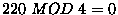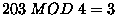Next: Operators Up: Grammatical Evolution Previous: Grammatical Evolution

## Example Individual

Consider an individual made up of the following genes (expressed in decimal for clarity) :

 220 203 17 3 109 215 104 30

These numbers will be used to look up the table in Section 2 which describes the BNF grammar for this particular problem. The first few rules don't involve any choice, so all individuals are of the form:

```float symb(float x)
{
a = <expr>;
return(a);
}
```

Concentrating on the `<expr>` part, we can see that there are four productions to choose from. To make this choice, we read the first gene from the chromosome, and use it to generate a protein in the form of a number. This number will then be used to decide which production rule to use, thus s we havewhich means we must take the first production, namely, 1A. We now have the following

```<expr> <op> <expr>
```

Notice that if this individual is subsequently wrapped, the first gene will still produce the protein 220. However, depending on previous proteins, we may well be examining the choice of another rule, possibly with a different amount of choices. In this way, although we have the same protein it results in a different physical trait.

Continuing with the first expression, a similar choice must be made, this time using, so the third choice is used, that is 1C.

```<var> <op> <expr>
```

There is no choice to be made for `var`, as there is only one possible outcome, so X, is inserted. Notice that no number is read from the genome this time.

```X <op> <expr>
```

The mapping continues, as summarized in table 3, until eventually, we are left with the following expression:

```X + Sin ( X )
```

Table 3: Mapping a genome onto an expression
 Expression Rule Gene Number Generated `` n/a `` 1A 220 0 `` 1D 203 3 `X` 4 n/a `X+` 2A 17 1 `X+pre_op()` 2C 19 3 `X+Sin()` 3A 109 1 `X+Sin()` 1D 215 3 `X+Sin(X)` 4 n/a

Notice how not all of the genes were required, in this case the extra genes are simply ignored.Next: Operators Up: Grammatical Evolution Previous: Grammatical Evolution
Red Hat Linux User
1998-10-02